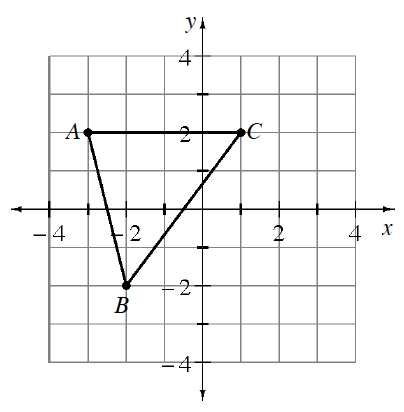### Home > CC2MN > Chapter 6 Unit 6A > Lesson CC3: 6.2.1 > Problem6-46

6-46.Louis is dilating triangle $ABC$ at right. He multiplied each $x$-coordinate and $y$-coordinate of triangle $ABC$ by $-2$.

1. What are the new coordinates of the points?

Use the graph to find the coordinates of points $A$, $B$, and $C$. Then multiply each value by $−2$. For example, point $A$ is originally $(−3,2)$. Its new coordinates will be $(6,−4)$. Find points $B$ and $C$.

2. Graph Louis’ new triangle.

After multiplying each $x$-coordinate and $y$-coordinate by $−2$, plot the new points.

3. Describe how triangle $ABC$ changed.

Its sides are twice as long and rotated $180°$.

Use the eTool below to graph Louis' new triangle.
Click the link at right for the full version of the eTool: CC3 6-46 HW eTool